Rank Correlation of Observed Responses and Predicted Probabilities

The predicted mean score of an observation is the sum of the Ordered Values (shown in the Response Profile table) minus one, weighted by the corresponding predicted probabilities for that observation; that is, the predicted means score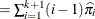, where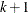is the number of response levels and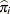is the predicted probability of the ith (ordered) response.

A pair of observations with different observed responses is said to be concordant if the observation with the lower ordered response value has a lower predicted mean score than the observation with the higher ordered response value. If the observation with the lower ordered response value has a higher predicted mean score than the observation with the higher ordered response value, then the pair is discordant. If the pair is neither concordant nor discordant, it is a tie. Enumeration of the total numbers of concordant and discordant pairs is carried out by categorizing the predicted mean score into intervals of length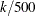and accumulating the corresponding frequencies of observations. Note that the length of these intervals can be modified by specification of the BINWIDTH= option in the MODEL statement.

Let N be the sum of observation frequencies in the data. Suppose there are a total of t pairs with different responses: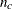of them are concordant,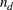of them are discordant, and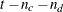of them are tied. PROC LOGISTIC computes the following four indices of rank correlation for assessing the predictive ability of a model: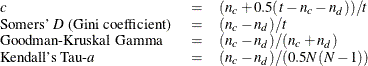If there are no ties, then Somers’ D (Gini’s coefficient)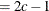. Note that the concordance index, c, also gives an estimate of the area under the receiver operating characteristic (ROC) curve when the response is binary (Hanley and McNeil, 1982). See the section ROC Computations for more information about this area.

For binary responses, the predicted mean score is equal to the predicted probability for Ordered Value 2. As such, the preceding definition of concordance is consistent with the definition used in previous releases for the binary response model.

These statistics are not available when the STRATA statement is specified.